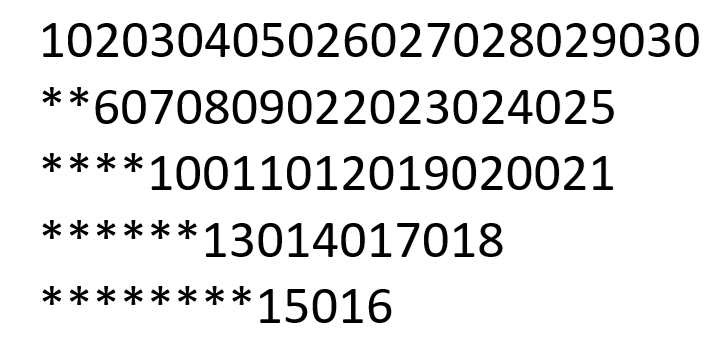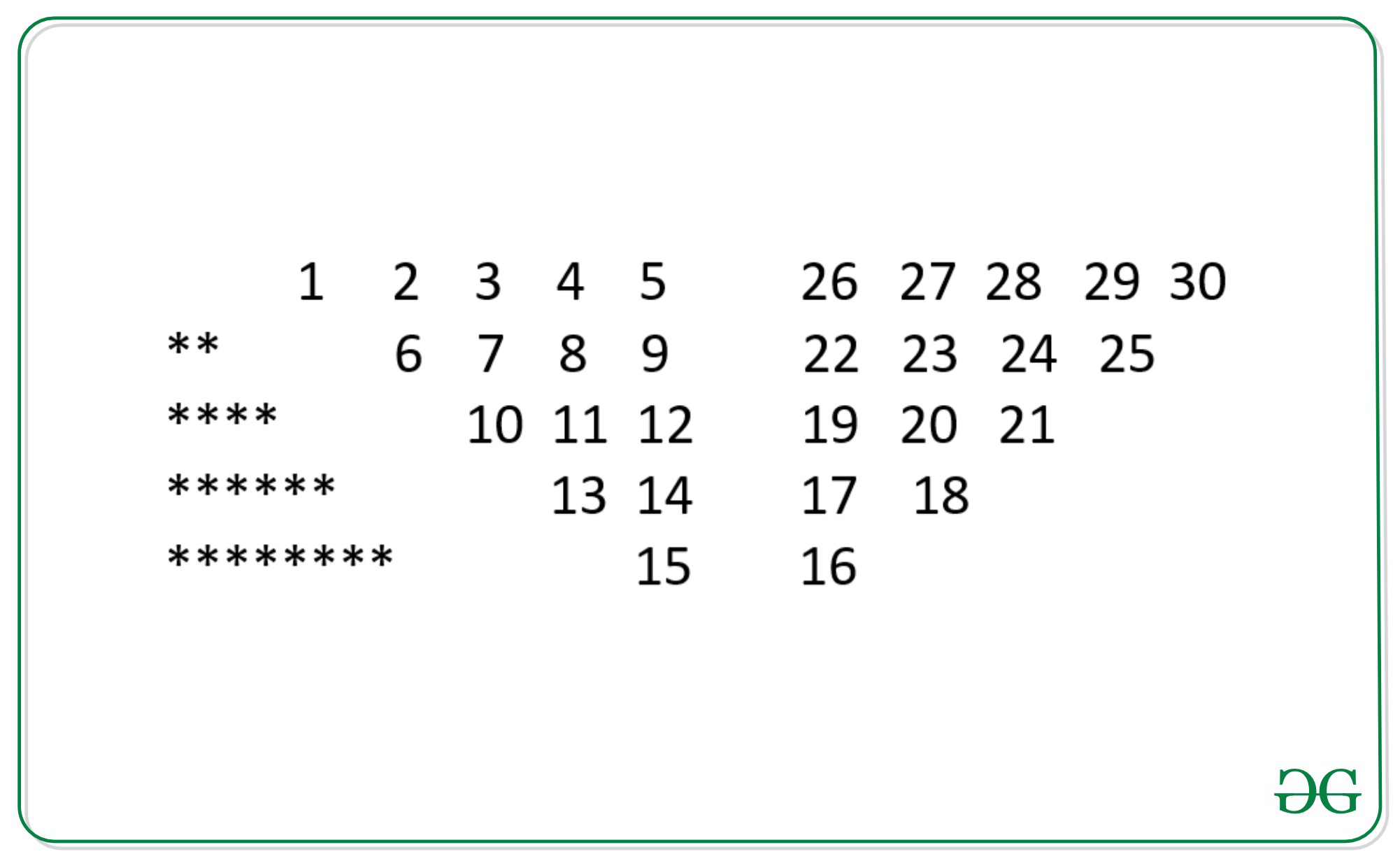# Program to print the pattern 1020304017018019020 **50607014015016 ****809012013 ******10011…

• Difficulty Level : Medium
• Last Updated : 21 May, 2021

Given an integer N, the task is to print the pattern below for the given value of N.

For N = 5, below is the given pattern:Examples:

Input: N = 4
Output:
1020304017018019020
**50607014015016
****809012013
******10011

Input: N = 3
Output:
10203010011012
**4050809
****607

Approach: The idea to understand the logic behind the given pattern is stated below:

By looking closely, we see that by replacing in-between zeroes with spaces, the pattern can be seen more clearly. The pattern is further divided into three different patterns.

1. Case 1: Asterisk (*) character pattern follows a sequence from 0 and adds two more asterisks in each row, where the row is equal to N.
2. Case 2: In this part, the pattern is very simple to understand. i.e the number of columns and rows will be equal to N and follows a sequence like 1, 2, 3, 4, 5…
3. Case 3: Follow-up or bottom-up sequence is the interesting part where the numbers are represented from bottom to top.Below is the implementation of the above approach:

## C++

 `// C++ implementation to print``// the given pattern``#include ``using` `namespace` `std;` `// Function to find the sum of``// N integers from 1 to N``int` `sum(``int` `n)``{``    ``return` `n * (n - 1) / 2;``}` `// Function to print the given``// pattern``void` `BSpattern(``int` `N)``{``    ``int` `Val = 0, Pthree = 0,``        ``cnt = 0, initial;` `    ``string s = ``"**"``;` `    ``// Iterate over [0, N - 1]``    ``for` `(``int` `i = 0; i < N; i++) {``        ``cnt = 0;` `        ``// Sub-Pattern - 1``        ``if` `(i > 0) {``            ``cout << s;``            ``s += ``"**"``;``        ``}` `        ``// Sub-Pattern - 2``        ``for` `(``int` `j = i; j < N; j++) {` `            ``// Count the number of element``            ``// in rows and sub-pattern 2 and 3``            ``// will have same rows``            ``if` `(i > 0) {``                ``cnt++;``            ``}``            ``// Increment Val to print the``            ``// series 1, 2, 3, 4, 5 ...``            ``cout << ++Val;``            ``cout << 0;``        ``}` `        ``// To get the first element of sub``        ``// pattern 3 find the sum of first N-1``        ``// elements first N-1 elements in row1``        ``// previous of Sub-Pattern 2` `        ``// Finally, add the (N-1)th element``        ``// i.e., 5 and increment it by 1``        ``if` `(i == 0) {``            ``int` `Sumbeforelast = sum(Val) * 2;``            ``Pthree = Val + Sumbeforelast + 1;``            ``initial = Pthree;``        ``}` `        ``// Initial is used to give the initial``        ``// value of the row in Sub-Pattern 3``        ``initial = initial - cnt;` `        ``Pthree = initial;` `        ``// Sub-Pattern 3``        ``for` `(``int` `k = i; k < N; k++) {` `            ``cout << Pthree++;` `            ``// Skip printing zero at the last``            ``if` `(k != N - 1) {``                ``cout << 0;``            ``}``        ``}` `        ``cout << ``"\n"``;``    ``}``}` `// Driver Code``int` `main()``{``    ``// Given N``    ``int` `N = 5;` `    ``// Function Call``    ``BSpattern(N);``    ``return` `0;``}`

## Java

 `// Java implementation to print``// the given pattern``import` `java.util.*;` `class` `GFG{` `// Function to find the sum of``// N integers from 1 to N``static` `int` `sum(``int` `n)``{``    ``return` `n * (n - ``1``) / ``2``;``}` `// Function to print the given``// pattern``static` `void` `BSpattern(``int` `N)``{``    ``int` `Val = ``0``, Pthree = ``0``,``        ``cnt = ``0``, initial = -``1``;` `    ``String s = ``"**"``;` `    ``// Iterate over [0, N - 1]``    ``for``(``int` `i = ``0``; i < N; i++)``    ``{``        ``cnt = ``0``;``        ` `        ``// Sub-Pattern - 1``        ``if` `(i > ``0``)``        ``{``            ``System.out.print(s);``            ``s += ``"**"``;``        ``}` `        ``// Sub-Pattern - 2``        ``for``(``int` `j = i; j < N; j++)``        ``{``            ` `            ``// Count the number of element``            ``// in rows and sub-pattern 2``            ``// and 3 will have same rows``            ``if` `(i > ``0``)``            ``{``                ``cnt++;``            ``}``            ` `            ``// Increment Val to print the``            ``// series 1, 2, 3, 4, 5 ...``            ``System.out.print(++Val);``            ``System.out.print(``"0"``);``        ``}` `        ``// To get the first element of sub``        ``// pattern 3 find the sum of first N-1``        ``// elements first N-1 elements in row1``        ``// previous of Sub-Pattern 2` `        ``// Finally, add the (N-1)th element``        ``// i.e., 5 and increment it by 1``        ``if` `(i == ``0``)``        ``{``            ``int` `Sumbeforelast = sum(Val) * ``2``;``            ``Pthree = Val + Sumbeforelast + ``1``;``            ``initial = Pthree;``        ``}` `        ``// Initial is used to give the initial``        ``// value of the row in Sub-Pattern 3``        ``initial = initial - cnt;` `        ``Pthree = initial;` `        ``// Sub-Pattern 3``        ``for``(``int` `k = i; k < N; k++)``        ``{``            ``System.out.print(Pthree++);` `            ``// Skip printing zero at the last``            ``if` `(k != N - ``1``)``            ``{``                ``System.out.print(``"0"``);``            ``}``        ``}``        ``System.out.println();``    ``}``}` `// Driver code``public` `static` `void` `main(String[] args)``{``    ` `    ``// Given N``    ``int` `N = ``5``;``    ` `    ``// Function call``    ``BSpattern(N);``}``}` `// This code is contributed by offbeat`

## Python3

 `# Python3 implementation to print``# the given pattern` `# Function to find the sum of``# N integers from 1 to N``def` `sum``(n):``    ` `    ``return` `n ``*` `(n ``-` `1``) ``/``/` `2`` ` `# Function to print the given``# pattern``def` `BSpattern(N):``    ` `    ``Val ``=` `0``    ``Pthree ``=` `0``,``    ``cnt ``=` `0` `    ``initial ``=` `-``1``    ``s ``=` `"**"`` ` `    ``# Iterate over [0, N - 1]``    ``for` `i ``in` `range``(N):``        ``cnt ``=` `0`` ` `        ``# Sub-Pattern - 1``        ``if` `(i > ``0``):``            ``print``(s, end ``=` `"")``            ``s ``+``=` `"**"``         ` `        ``# Sub-Pattern - 2``        ``for` `j ``in` `range``(i, N):``            ` `            ``# Count the number of element``            ``# in rows and sub-pattern 2 and 3``            ``# will have same rows``            ``if` `(i > ``0``):``                ``cnt ``+``=` `1``             ` `            ``# Increment Val to print the``            ``# series 1, 2, 3, 4, 5 ...``            ``Val ``+``=` `1``            ``print``(Val, end ``=` `"")``            ``print``(``0``, end ``=` `"")``         ` `        ``# To get the first element of sub``        ``# pattern 3 find the sum of first N-1``        ``# elements first N-1 elements in row1``        ``# previous of Sub-Pattern 2`` ` `        ``# Finally, add the (N-1)th element``        ``# i.e., 5 and increment it by 1``        ``if` `(i ``=``=` `0``):``            ``Sumbeforelast ``=` `sum``(Val) ``*` `2``            ``Pthree ``=` `Val ``+` `Sumbeforelast ``+` `1``            ``initial ``=` `Pthree``         ` `        ``# Initial is used to give the initial``        ``# value of the row in Sub-Pattern 3``        ``initial ``=` `initial ``-` `cnt`` ` `        ``Pthree ``=` `initial`` ` `        ``# Sub-Pattern 3``        ``for` `k ``in` `range``(i, N):``            ``print``(Pthree, end ``=` `"")``            ``Pthree ``+``=` `1`` ` `            ``# Skip printing zero at the last``            ``if` `(k !``=` `N ``-` `1``):``                ``print``(``0``, end ``=` `"")``                ` `        ``print``()``        ` `# Driver Code` `# Given N``N ``=` `5`` ` `# Function call``BSpattern(N)` `# This code is contributed by sanjoy_62`

## C#

 `// C# implementation to print``// the given pattern``using` `System;``class` `GFG{`` ` `// Function to find the sum of``// N integers from 1 to N``static` `int` `sum(``int` `n)``{``    ``return` `n * (n - 1) / 2;``}`` ` `// Function to print the given``// pattern``static` `void` `BSpattern(``int` `N)``{``    ``int` `Val = 0, Pthree = 0,``        ``cnt = 0, initial = -1;`` ` `    ``String s = ``"**"``;`` ` `    ``// Iterate over [0, N - 1]``    ``for``(``int` `i = 0; i < N; i++)``    ``{``        ``cnt = 0;``         ` `        ``// Sub-Pattern - 1``        ``if` `(i > 0)``        ``{``            ``Console.Write(s);``            ``s += ``"**"``;``        ``}`` ` `        ``// Sub-Pattern - 2``        ``for``(``int` `j = i; j < N; j++)``        ``{``             ` `            ``// Count the number of element``            ``// in rows and sub-pattern 2``            ``// and 3 will have same rows``            ``if` `(i > 0)``            ``{``                ``cnt++;``            ``}``             ` `            ``// Increment Val to print the``            ``// series 1, 2, 3, 4, 5 ...``            ``Console.Write(++Val);``            ``Console.Write(``"0"``);``        ``}`` ` `        ``// To get the first element of sub``        ``// pattern 3 find the sum of first N-1``        ``// elements first N-1 elements in row1``        ``// previous of Sub-Pattern 2`` ` `        ``// Finally, add the (N-1)th element``        ``// i.e., 5 and increment it by 1``        ``if` `(i == 0)``        ``{``            ``int` `Sumbeforelast = sum(Val) * 2;``            ``Pthree = Val + Sumbeforelast + 1;``            ``initial = Pthree;``        ``}`` ` `        ``// Initial is used to give the initial``        ``// value of the row in Sub-Pattern 3``        ``initial = initial - cnt;`` ` `        ``Pthree = initial;`` ` `        ``// Sub-Pattern 3``        ``for``(``int` `k = i; k < N; k++)``        ``{``            ``Console.Write(Pthree++);`` ` `            ``// Skip printing zero at the last``            ``if` `(k != N - 1)``            ``{``                ``Console.Write(``"0"``);``            ``}``        ``}``        ``Console.WriteLine();``    ``}``}`` ` `// Driver code``public` `static` `void` `Main(String[] args)``{``     ` `    ``// Given N``    ``int` `N = 5;``     ` `    ``// Function call``    ``BSpattern(N);``}``}`` ` `// This code is contributed by shikhasingrajput`

## Javascript

 ``

Output:

```102030405026027028029030
**6070809022023024025
****10011012019020021
******13014017018
********15016```

Time Complexity: O(N2)
Auxiliary Space: O(1)

My Personal Notes arrow_drop_up